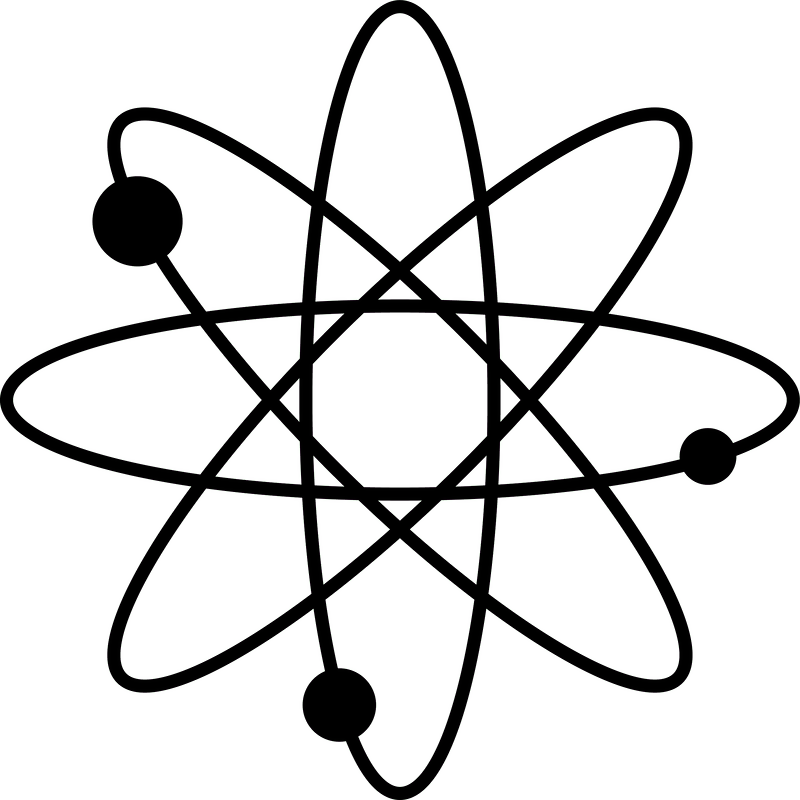﻿Physics Primer

Nuclear Reactions

Let's start with a very basic recap of chemistry and atomic physics:

atoms are made out of a positively charged nucleus (protons + neutrons) and a negatively charged electron cloud (electrons) 原子由带正电的原子核（质子+中子）和带负电荷的电子云（电子）组成。

a chemical element is identified by its number of protons which we generally refer to as the atomic number (Z). E.g. Hydrogen has one proton, and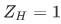; Oxygen has eight protons, and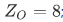; Uranium has 92 protons, and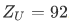the sum of the number of protons and neutrons in a nucleus is generally called nucleon number or atomic mass number (A). Because the proton and neutron masses are very close to each other (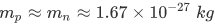), and the electron mass is negligible compared to the proton mass (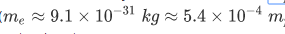), the total mass of an atom can be approximated to the atomic mass number x the mass of one proton/neutron.

a useful quantity is the unified atomic mass unit (u) that is approximately equal to the mass of one proton/neutron: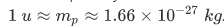. Then the mass of some element X is going to be mX=AX×u. E.g.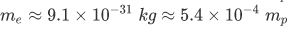. As we will see, this is not quite true. The mass of an element is not just the mass of its components; instead one must also include the mass equivalent for the binding energy between the protons and neutrons. 

two elements with the same number of protons, but different number of neutrons are called isotopes. E.g. U-238 and U-235 have 92 protons, but 146 and respectively 143 neutrons.

we are going to write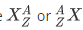when referring to an element X with atomic mass number A and atomic number Z. E.g.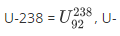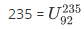.

we are often going to write down nuclear reactions such as: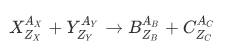For each nuclear reaction we can write down two equalities: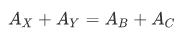，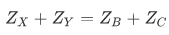.

These two equalities impose the conservation of mass number (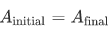) and conservation of charge (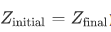）.

E.g.: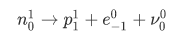In this nuclear reaction a neutron decays into a proton and two extra particles (an electron and a neutrino). The overall charge and atomic mass number are conserved.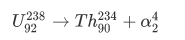Uranium-238 decays into Thorium plus an alpha-particle (2 protons and 2 electrons). 铀-238衰变为钍加一个α粒子（2个质子和2个电子）。

for a nuclear reaction to be correct, atomic mass number and charge must be conserved. However, not all correct nuclear reactions are possible or likely. Factors like binding energy, half-life, stability, kinetic energy etc. can play an essential role in favoring some reactions over others.